# Multiplication by 1 Digit Number

## NUMBERS AND OPERATIONSThe multiplication is that operation that consists of calculating the product (result) that is obtained by adding the same number (factor or multiplicand) so many times as the value of the other number (factor or multiplicator or multiplier).

Multiplication is used when we have to replace the calculation of certain repetitive additions (for example: 2 + 2 + 2 + 2 = 8) by a more efficient method (2 x 4 = 8).

In general, multiplication is considered the inverse operation of division.

MULTIPLY TABLES FROM 1 TO 10

We have prepared a template with the multiplication tables from 1 to 10 ready to download and print so that you can consult them when you need it and help you learn them quickly.

PYTHAGORAS MULTIPLY TABLES

We have also prepared a complete Pythagorean table where the multiplication tables from 1 to 10 are represented to facilitating very visual learning using patterns and symmetries and stimulate logical reasoning among students.

#### Know the terms of multiplication

Do you know what multiplications are and what are they for? Can you tell what its symbol "x" means? Do you know the differences between the multiplicand, the multiplier and the product ? Don't worry, through the lesson we explain each of the elements of multiplication.

SPANISHENGLISHThe elements of multiplication (multiplicand and multiplier) that are multiplied together are called "factors" or "coefficients":

- The multiplicand is the element that tells us "how many times" we are going to add or multiply the multiplier digit (in this example, it is 24). It is at the top of any multiplication.

- The multiplier represents the element that multiplies each of the numbers in the multiplicand and we will take as a reference its multiplication table (in this case, the table of 7). It is located at the bottom of the multiplication and is always written to the right of the multiplication sign ("x")

- The multiplication sign determines the multiplicative operation and is read "multiplied by" or "times", in the following example, it would be "24 multiplied by 7" or "24 times 7". It is represented by a cross "x" (relative to the Cross of Saint Andrew) or by using a point at half height (•)

- The equal sign is the equality symbol and is read "equals". In our example, we will read the multiplication as "24 times 7 equals 168"

- The product contains the result of applying the multiplication algorithm (24 x 7 = 168)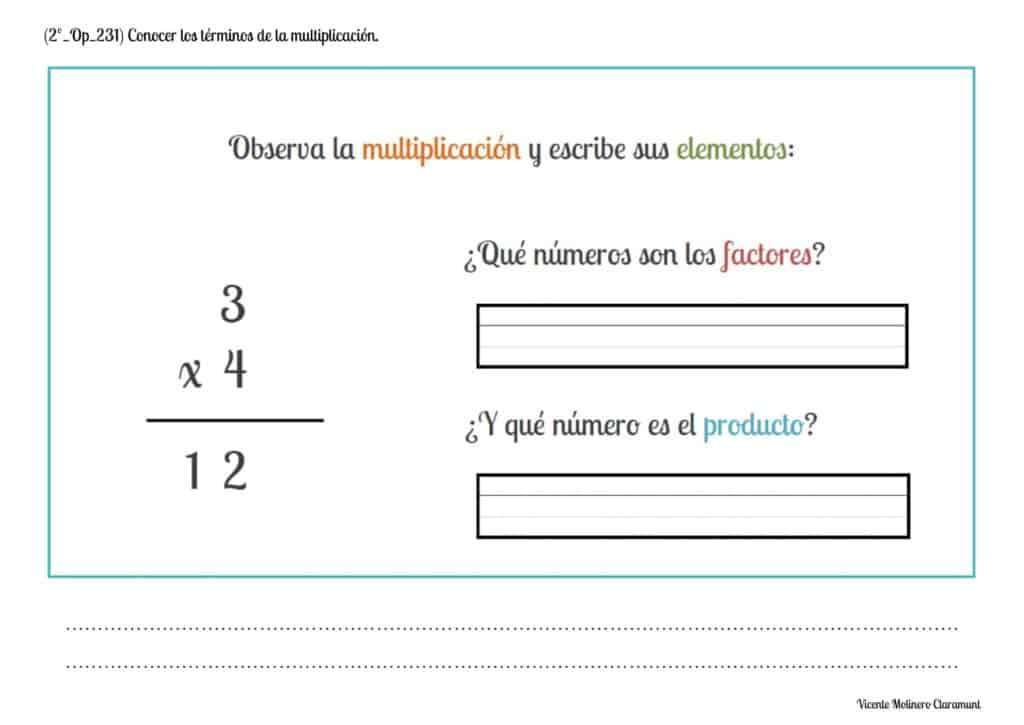#### Recognize the concept of multiplication as a repeated addition of equal addends

We must understand that, mathematically, multiplying a certain quantity by a number consists of adding said quantity as many times as the number indicates.

In other words, multiplications are those mathematical operations that are carried out to calculate the result (also called "product") of adding the same number ("multiplicand") so many times as indicated by the other number ("multiplier").

We present you some printable PDF activities accompanied by their explanation and an educational game so that you understand that all multiplication is equivalent to the repeated addition of equal addends.

SPANISHENGLISHIn the following example we can see that multiplying 5 times 3 (that is 5x3) is also equivalent to adding 3 times the number 5 (meaning 5+5+5), obtaining in both cases the same result (product):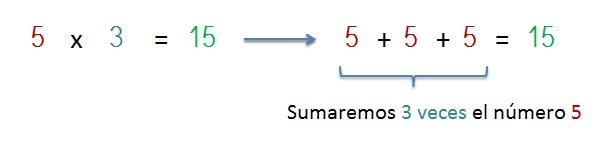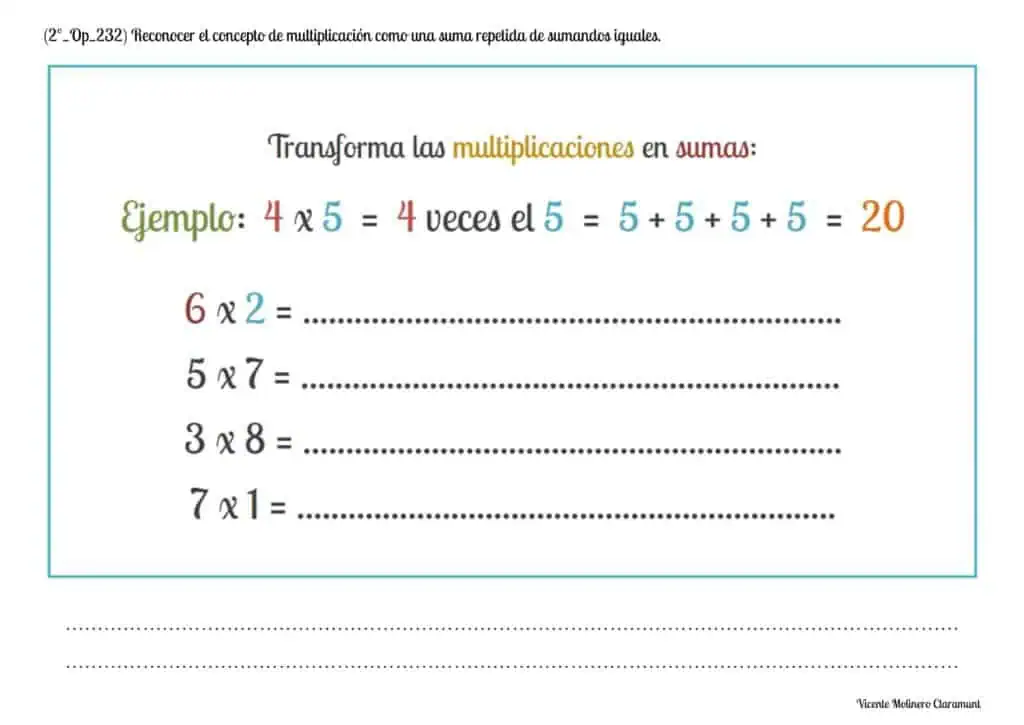#### Multiply natural numbers: 1 digit by 1 digit

We propose some printable exercises accompanied by their explanation and an educational game so that you can practice and learn the algorithm of the multiplication of a digit and its vertical resolution.

SPANISHENGLISHUsing a worked example, we are going to teach you, step by step, how to solve any multiplication of a number in the multiplicand by a number in the multiplier: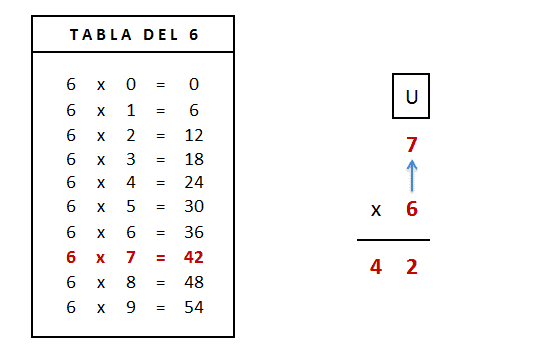To find the result ("product") of a multiplication of a figure by a figure, we will take as reference the multiplication table of the multiplier (Table of 6).

Next, we will multiply the number of the multiplier (6) by the number of the multiplicand (7) consulting its numerical value in the Table of 6, that is 6 x 7 = 42

Finally, we will annotate the result (42) below the horizontal dividing line.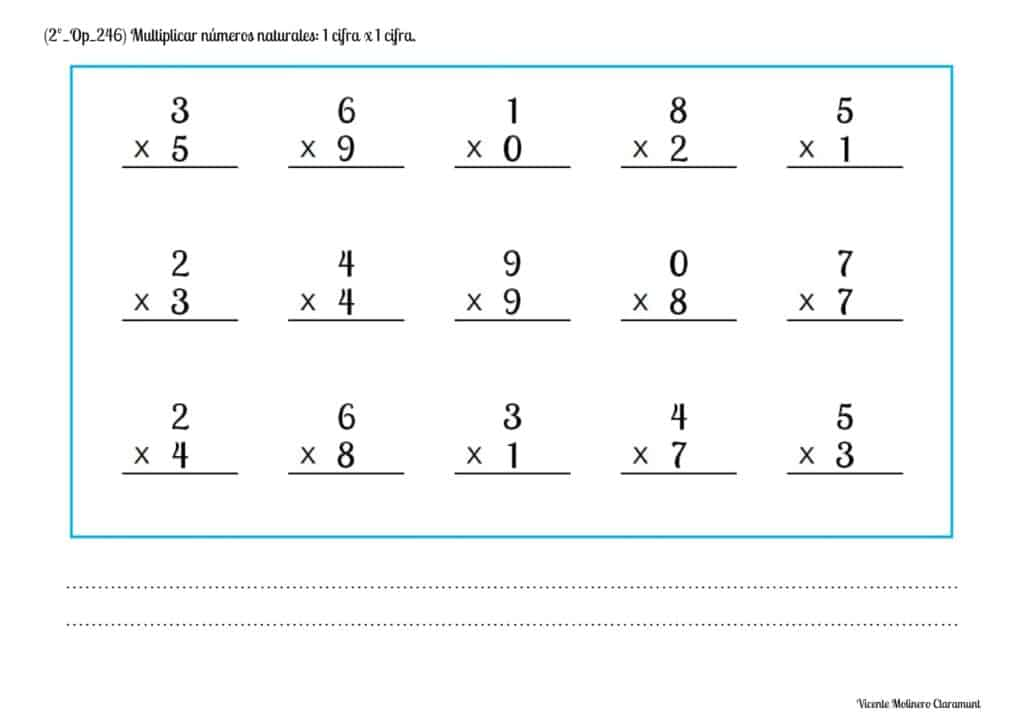#### Solve multiplication word problems with natural numbers: 1 digit by 1 digit

We have developed a set of multiplication problems by a number for you to learn to solve. In addition, we explain what is the process to find the solution from various solved examples.

SPANISHSPANISHENGLISH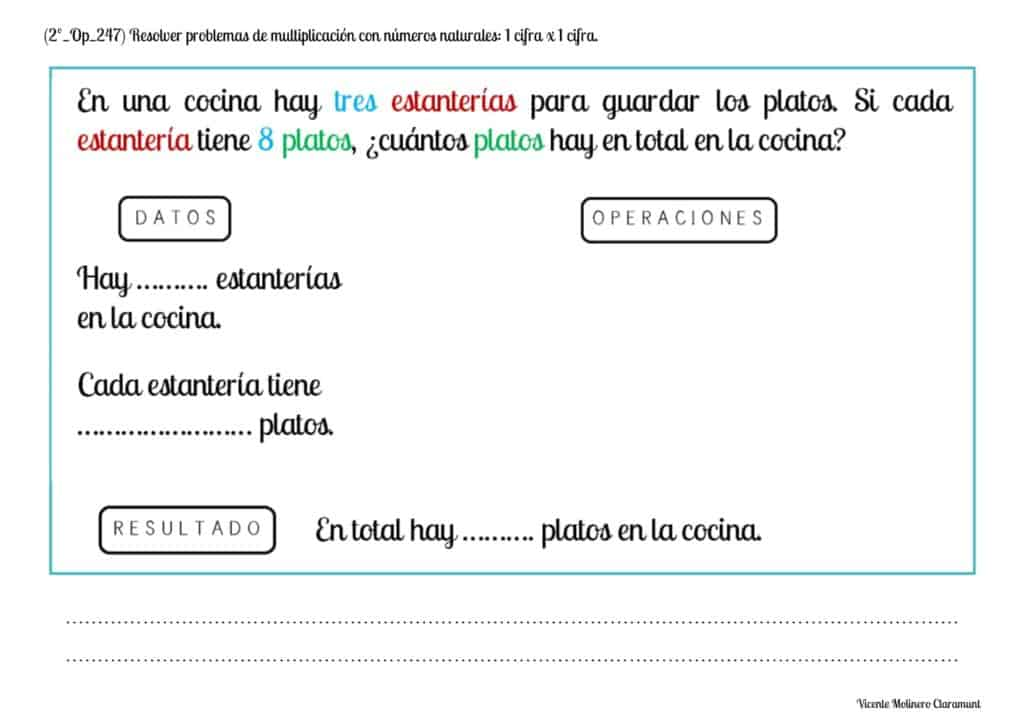#### Calculate double quantities

Do you know how to calculate double of something? You only need to use the multiplication table of 2 since double any quantity is found by multiplying any initial digit (multiplicand) by the number two (multiplier). As simple as that!

In the example that we propose we can see how to calculate the double of 2 elements, the resulting operation being 2 x 2 = 4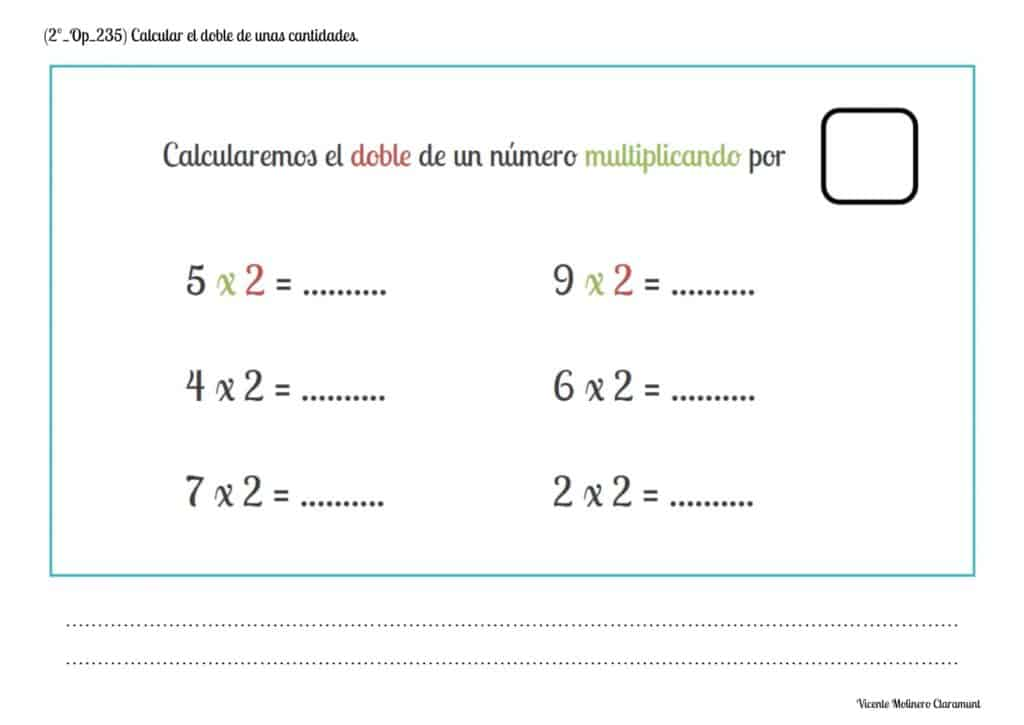#### Solve doubles word problems

After learning the Table of 2 and the concept of calculating double a quantity, we have developed a series of problems so that you can demonstrate your math skills.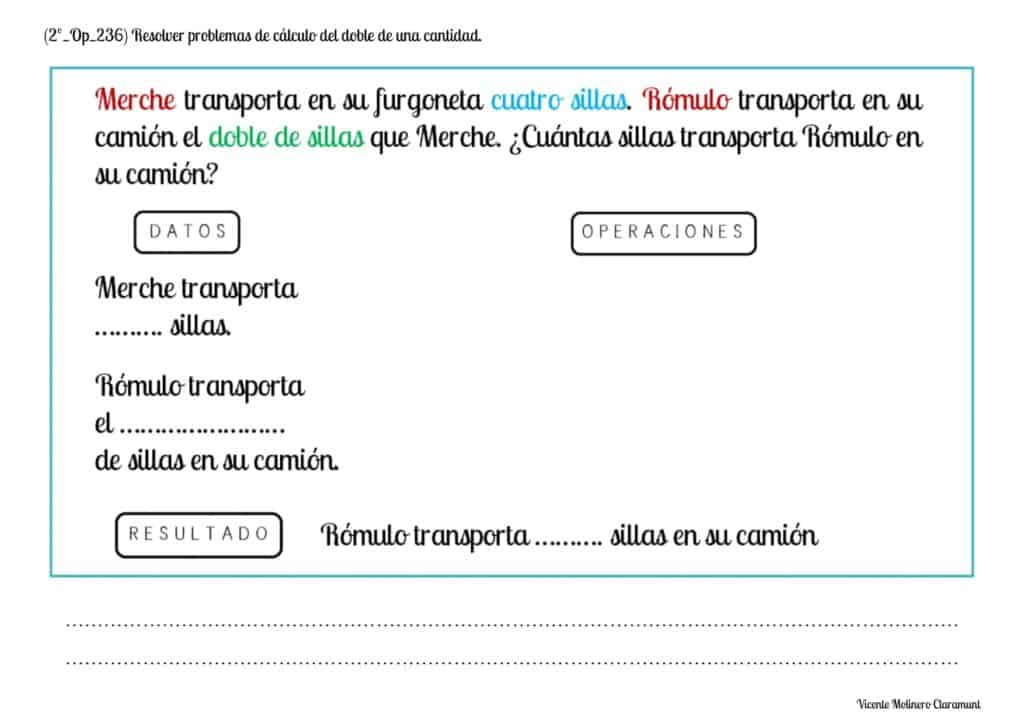#### Calculate triple quantities

Do you know how to calculate the triple of a number? You only need to use the multiplication table of 3 since the triple of any quantity is found by multiplying any initial figure (multiplicand) by the number three (multiplier). Easy peasy!

In the example that we provide, we can see how to calculate the triple of 2 elements, the resulting operation being 2 x 3 = 6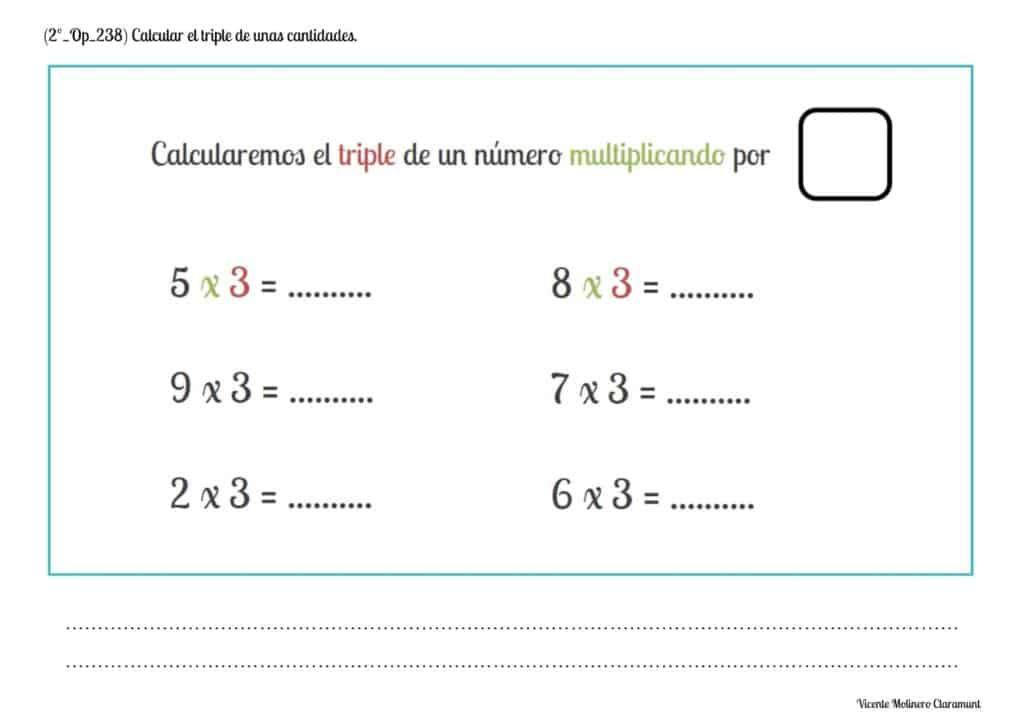#### Solve triples word problems

After learning the Table of 3 and the concept of calculating the triple of a quantity, we have developed a variety of problems so that you can develop your mathematical reasoning.#### Multiply natural numbers without carrying: 2 digits by 1 digit

We present you some educational sheets in PDF format accompanied by their explanation and an online game so that you understand step by step how to solve two-digit multiplications for a number without carry.

SPANISHENGLISHThrough a worked example, you will learn how to calculate a multiplication of two digits in the multiplicand by one digit in the multiplier and no carrying:Let's multiply 43 x 2

1st) We start by multiplying the multiplier (lower digit) by the number of the ones of the multiplicand (upper digit):

2 x 3 = 6 ones

2nd) Next, we will write the result of the multiplication (6) below the horizontal dividing line and aligning it with the figure of the ones.

3rd) We will multiply, on this occasion, the figure of the multiplier (table of 2) by the figure of the tens of the multiplicand:

2 x 4 = 8 tens

4th) Finally, we write the result of the multiplication (8) below the figure of the tens and after the horizontal dividing line.

You have already solved the multiplication! The result: 43 x 2 = 86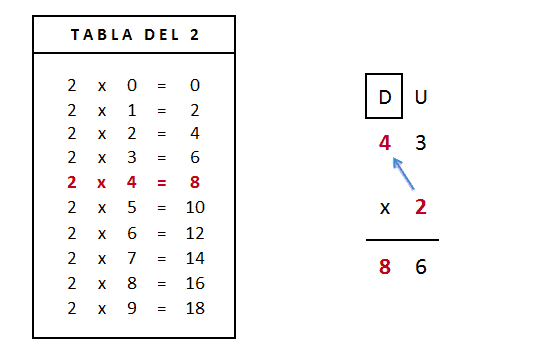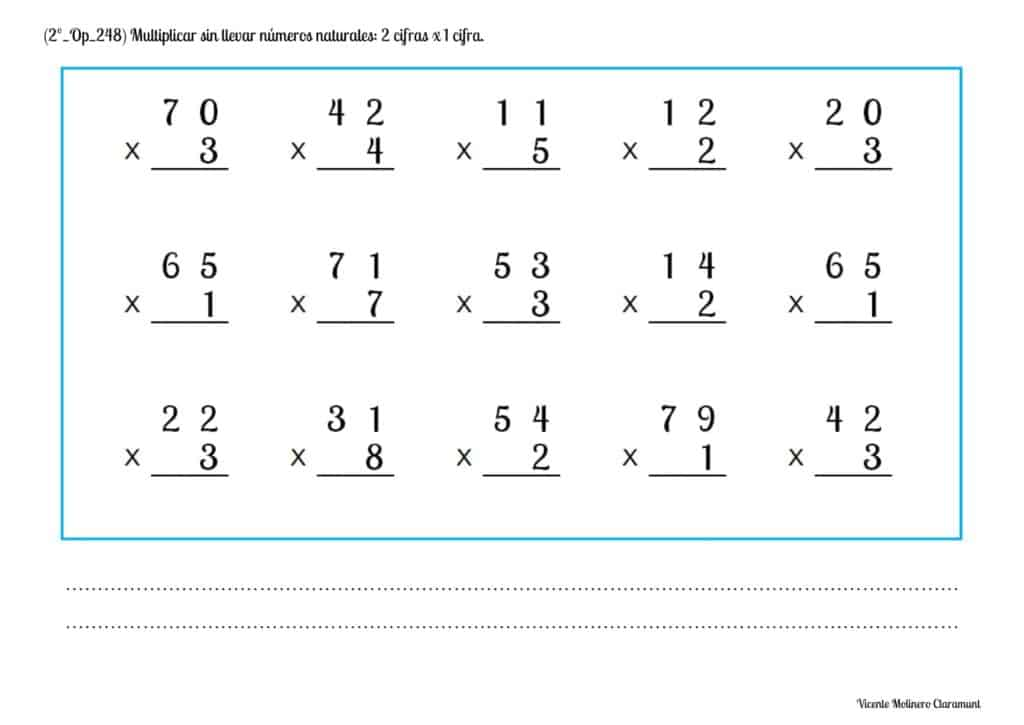#### Solve multiplication word problems without carrying with natural numbers: 2 digits x 1 digit

We offer you a series of multiplication word problems of two figures by one figure (without regrouping) for you to continue improving. We also provide you with video lessons where we explain in a simple way what is the procedure for its resolution. Time to practice!

SPANISHSPANISHENGLISH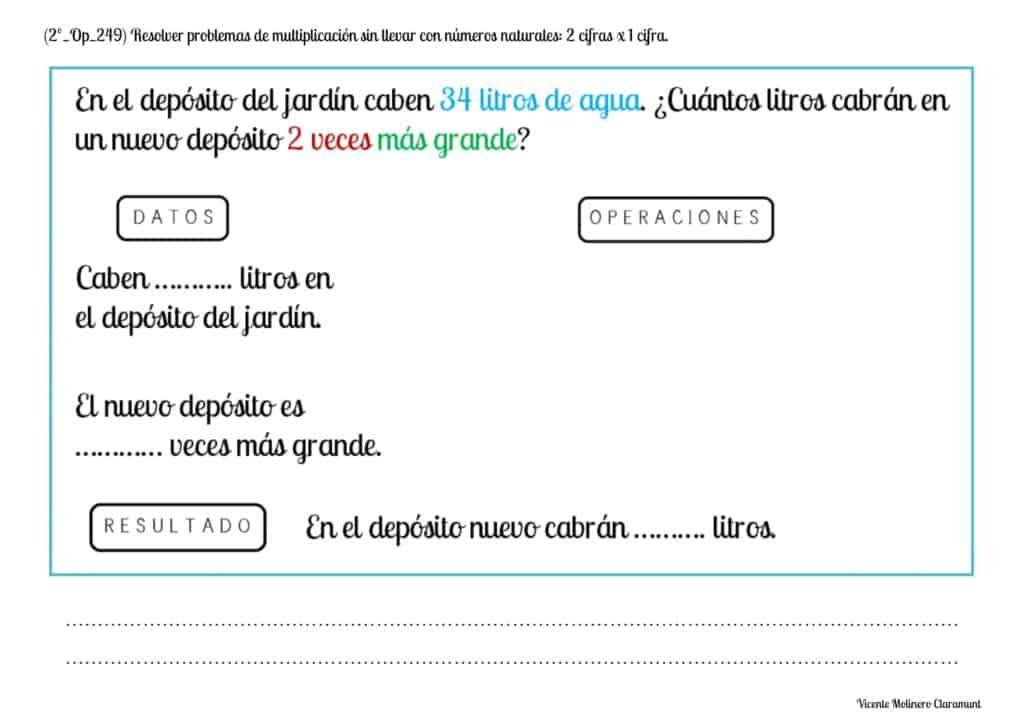#### Multiply natural numbers with carrying: 2 digits by 1 digit

We present you some educational worksheets ready to download accompanied by their explanation and an interactive activity so that you can learn to solve, in simple steps, the multiplication of two figures by one figure and regrouping.

SPANISHENGLISHThrough the example that we propose, you will learn how to calculate a multiplication of two digits in the multiplicand by one digit in the multiplier and carrying: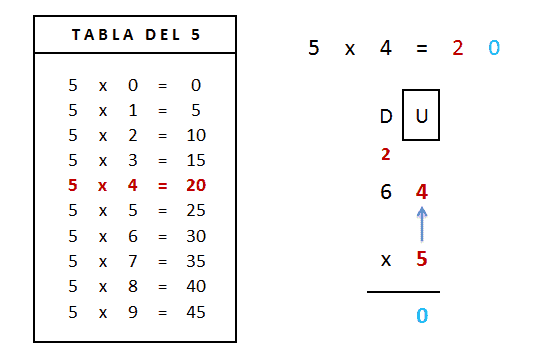Let's multiply 64 x 5

1st) We start by multiplying the multiplier (lower digit) by the number of the ones of the multiplicand (upper digit):

5 x 4 = 20 ones

2nd) The 20 represents 2 tens and 0 ones. We will align the digit 0 below the dividing line (since it corresponds to the ones) and we will save the 2 tens in the tens column to add them in the next step.

3rd) We will multiply, on this occasion, the figure of the multiplier (table of 5) by the figure of the tens of the multiplicand:

5 x 6 = 30 tens

We will add to the result the 2 tens that we had previously saved:

30 + 2 = 32

4th) Finally, we write the result of the multiplication (32) below the figure of the tens and after the horizontal dividing line.

You have already solved the multiplication! The result: 64 x 5 = 320#### Solve multiplication word problems with carrying with natural numbers: 2 digits x 1 digit

We propose you our multiplication word problems of two figures by one figure (regrouping) so that you continue to improve. In addition, we provide you with video lessons where we explain in a practical way what is the procedure for its correct resolution.

SPANISHSPANISHENGLISH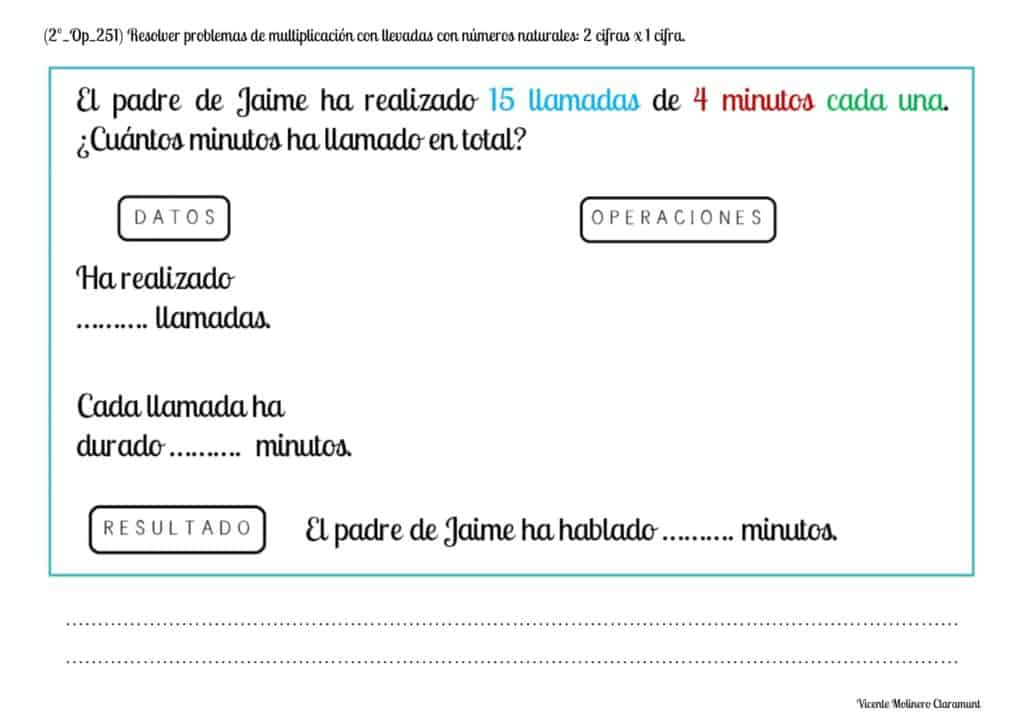#### Mentally calculate simple operations: Multiplication

Here are some mental math activities to test your memory and show that you already know all the multiplication tables.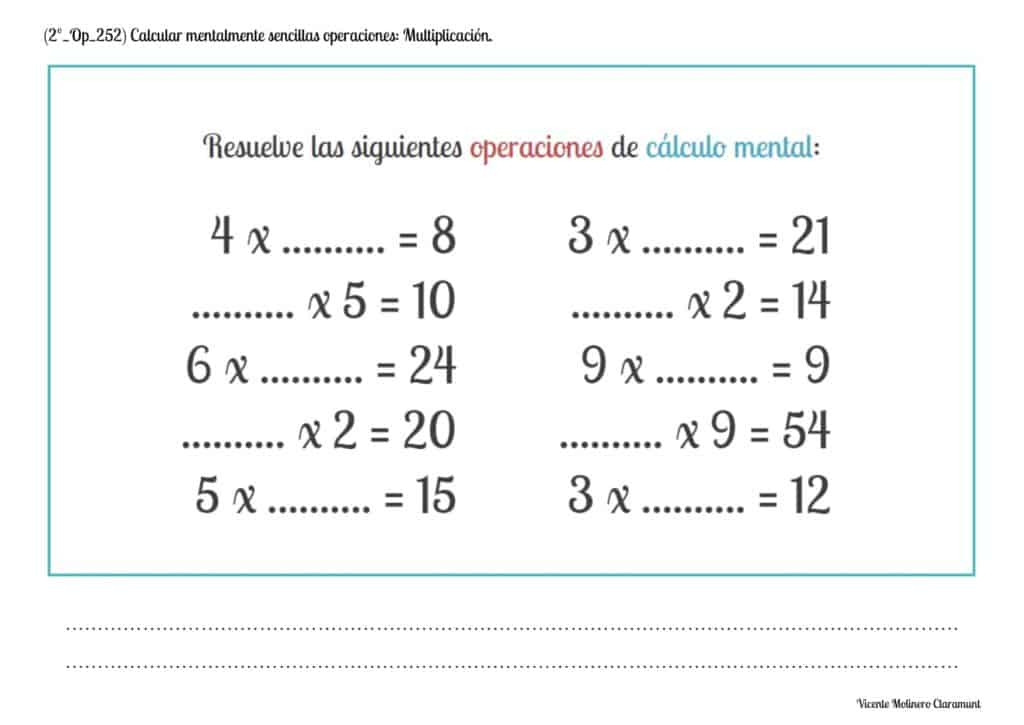Select more exercises, worksheets and activities of Mathematics for each of the 4 learning blocks of the Educational Curriculum ("Numbers and Operations", "Quantities and Measurements", "Geometric and Spatial Reasoning" and "Data Analysis and Probability") aimed at improving the logical-mathematical competencies and skills that are developed throughout "Grade 2" or "Year 3":